Quantitative Aptitude - Test 14

# Quantitative Aptitude - Test 14

Test Description

## 25 Questions MCQ Test SSC CGL Tier 1 Mock Test Series | Quantitative Aptitude - Test 14

Quantitative Aptitude - Test 14 for SSC CGL 2023 is part of SSC CGL Tier 1 Mock Test Series preparation. The Quantitative Aptitude - Test 14 questions and answers have been prepared according to the SSC CGL exam syllabus.The Quantitative Aptitude - Test 14 MCQs are made for SSC CGL 2023 Exam. Find important definitions, questions, notes, meanings, examples, exercises, MCQs and online tests for Quantitative Aptitude - Test 14 below.
Solutions of Quantitative Aptitude - Test 14 questions in English are available as part of our SSC CGL Tier 1 Mock Test Series for SSC CGL & Quantitative Aptitude - Test 14 solutions in Hindi for SSC CGL Tier 1 Mock Test Series course. Download more important topics, notes, lectures and mock test series for SSC CGL Exam by signing up for free. Attempt Quantitative Aptitude - Test 14 | 25 questions in 15 minutes | Mock test for SSC CGL preparation | Free important questions MCQ to study SSC CGL Tier 1 Mock Test Series for SSC CGL Exam | Download free PDF with solutions
 1 Crore+ students have signed up on EduRev. Have you?
Quantitative Aptitude - Test 14 - Question 1

### Directions: The following pie chart shows the marks scored by a student in different subjects- viz. Physics (Ph), Chemistry (Ch), Mathematics (M), Social Science (SS) and English (E) in an examination. Assuming that total marks obtained for the examination is 810, answer the questions given below. The marks obtained in English, Physics and Social Science exceed the marks obtained in Mathematics and Chemistry by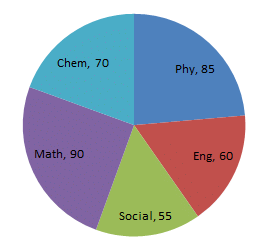The difference of marks between Physics and Chemistry is same as that between

Detailed Solution for Quantitative Aptitude - Test 14 - Question 1

As we know share of marks will be proportional to share of angles in pie chart
Hence Difference of marks in physics and chemistry is proportional to 15
and difference of marks in chemistry and social science is also proportional to 15 too.

Quantitative Aptitude - Test 14 - Question 2

###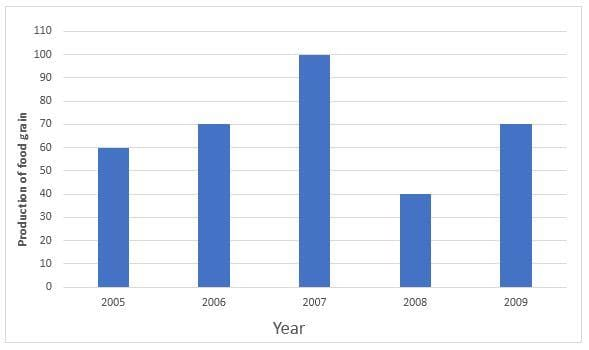Study the above bar graph showing the production of food grains (in million tons). What is the ratio between the maximum production and the minimum production during the given period? Graph should be drawn

Detailed Solution for Quantitative Aptitude - Test 14 - Question 2

Clearly, in the year 2007, maximum production of 100 mil tonnes is observed.
While in the year 2008, minimum production of 40 mil tonnes is observed.
Hence, the ratio is 100 : 40 = 5 : 2

Quantitative Aptitude - Test 14 - Question 3

### Rs. 700 is divided among A, B, C in such a way that the ratio of the amounts of A and B is 2 : 3 and that of B and C is 4 : 5. Find the amounts in Rs. each received, in order A, B, C.

Detailed Solution for Quantitative Aptitude - Test 14 - Question 3

A : B = 2 : 3

B : C = 4 : 5

A : B : C = 8 : 12 : 15

amount received by A = 8/(8 + 12 + 15) * 700 = 160/-

amount received by B = 12/(8 + 12 + 15) * 700 = 240/-

amount received by C = 15/(8 + 12 + 15) * 700 = 300/-

so the answer is option B.

Quantitative Aptitude - Test 14 - Question 4

ABC is a right angled triangle, B being the right angle. Mid-points of BC and AC are respectively B’ and A’. The ratio of the area of the quadrilateral AA’ B’B to the area of the triangle ABC is

Detailed Solution for Quantitative Aptitude - Test 14 - Question 4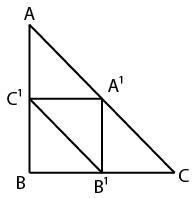let A', B', C' are the midpoints of AC, BC, AB.

areas of all 4 small triangles are same, let it be x.

area of quadrilateral AA'B'B = 3x

area of ABC = 4x

RATIO = 3x : 4x
= 3 : 4

so the answer is option C.

Quantitative Aptitude - Test 14 - Question 5

The ratio of the volumes of water and glycerine in 240cc of a mixture is 1 : 3. The quantity of water (in cc that should be added to the mixture so that the new ratio of the volume of water and glycerine becomes 2 : 3 is

Detailed Solution for Quantitative Aptitude - Test 14 - Question 5

Let's say we added x cc of water.
According to previous ratio amount of water in mixture is 240 × 1/4​ = 60
So after adding amount of water = 60 + x
amount of glycerine = 240 × 3/4 = 180
Hence new ratio will be =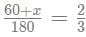or x will be 60.

Quantitative Aptitude - Test 14 - Question 6

If the distance between two point (0, -5) and (x, 0) is 13 unit, then x =

Detailed Solution for Quantitative Aptitude - Test 14 - Question 6

Distance2 = diff. of x cordinates2 + diff. of y coordinates2
132 = x2 + 52
Hence x = ±12

Quantitative Aptitude - Test 14 - Question 7

A and B together can do a works in 12 days. B and C together do it in 15 days. If A's efficiency is twice that of C, then the days required for B alone to finish the work is

Detailed Solution for Quantitative Aptitude - Test 14 - Question 7

A and B will do in 1 day, 1/12​ amount of work
B and C will do in 1 day, 1/15​ amount of work
Hence adding up above two equations
A + 2B + C will do in 1 day, 9/60 amount of work
as it is mentioned A = 2C (i.e. efficiency of A is twice of C)
So 2C + 2B + C will do in 1 day 9/60 amount of work
or 2(B+C) + C = 9/60
or C = 1/60​ (as B + C = 1/15)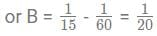So B alone will do work in 20 days.

Quantitative Aptitude - Test 14 - Question 8

A drum of kerosene is 3/4​ full. When 30 litres of kerosene is drawn from it, it remains 1/2​ full. The capacity of the drum is

Detailed Solution for Quantitative Aptitude - Test 14 - Question 8

Drum is 3/4​ full.
When 30 liters are drawn out of it, it becomes 1/2​ full.
Therefore 3/4 - 1/2​ of drum = 30
1/4 of drum = 30
Total capacity of drum = 30 × 4 = 120 litres

Quantitative Aptitude - Test 14 - Question 9

A rectangular sheet of metal is 40 cm by 15 cm. Equal squares of side 4 cm are cut off at the corners and the remainder is folded up to form an open rectangular box. The volume of the box is

Detailed Solution for Quantitative Aptitude - Test 14 - Question 9

After cutting off squares of sides 4cm. from rectangular sheet of 40cm × 15cm and making a rectangular box
New dimentions of rectangular will be:
Base = 32cm × 7cm
Height = 4 cm
Hence volume will be 32 × 7 × 4 = 896 cm3

Quantitative Aptitude - Test 14 - Question 10

Water flows into a tank which is 200m long and 150m wide, through a pipe of cross-section 0.3m × 0.2m at 20 km/hour. Then the time (in minutes) for the water level in the tank to reach 8cm is

Detailed Solution for Quantitative Aptitude - Test 14 - Question 10

Volume of water in time t to reach at 8 cm. height will be equal to volume of tank till height 8 cm.
hence volume of water in time t to reach at height 8 cm. = .3 × .2 × 2000/6 ​× (speed in per minute)
which is equal to volume of tank = 200 × 150 × 8/100​

equating both and after solving we will get t = 120 min.

Quantitative Aptitude - Test 14 - Question 11

5tanθ = 4, then the value of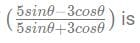Detailed Solution for Quantitative Aptitude - Test 14 - Question 11

Taking cosθ outside in numerator and in denominator and making tanθ
hence eq will be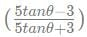As it is given that 5tanθ = 4
after putting values and solving we will get the equation reduced to 1/7.

Quantitative Aptitude - Test 14 - Question 12

The maximum value of sin θ + cos θ is

Detailed Solution for Quantitative Aptitude - Test 14 - Question 12

For asin θ + bcos θ + c,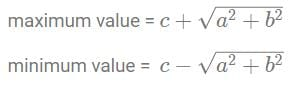for  sin θ + cos θ , a = 1, b = 1, c = 0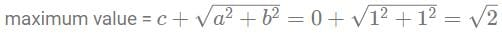so the answer is option B.

Quantitative Aptitude - Test 14 - Question 13

If x = cosecθ − sinθ and y = secθ − cosθ, then the value of x2y2(x2 + y2 + 3)

Detailed Solution for Quantitative Aptitude - Test 14 - Question 13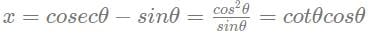Similarly y = sinθcosθ
x2 + y2 + 3 = (sec2θ + cosec2θ)
Now putting above values in given equation, and after solving it will be reduced to 1

Quantitative Aptitude - Test 14 - Question 14

The value of sin2 22° + sin2 68° + cot30° is

Detailed Solution for Quantitative Aptitude - Test 14 - Question 14

Expression: sin2 22° + sin2 68° + cot30°

We know that sin(90° − θ) = cosθ

=> sin22° = sin(90° − 68°) = cos68°

=> cos268° + sin268° + (√3​)2

=> 1 + 3 = 4

Quantitative Aptitude - Test 14 - Question 15

If the number of vertices, edges and faces of a rectangualr parallelopiped are denoted by v, e and f respectively, the value of (v - e + f) is

Detailed Solution for Quantitative Aptitude - Test 14 - Question 15

Euler's polyhedral formula states that the number of vertices V, faces F and edges E in a polyhedron satisfy:

V + F - E = 2

=> Ans - (B)

Quantitative Aptitude - Test 14 - Question 16

The average age of a jury of 5 is 40. If a member aged 35 resigns and man aged 25 becomes a member, then the average age of the new jury is

Detailed Solution for Quantitative Aptitude - Test 14 - Question 16

Initially, sum of ages of the jury = 200,
then man aged 35 resigns, sum of ages = 200 - 35
then man aged 25 joins, sum of ages = 200 - 35 + 25
Final number of members in the jury = 5
So, average age of the jury = (200 - 35 + 25)/5 = 38

Quantitative Aptitude - Test 14 - Question 17

I am three times as old as my son. 15 years hence, I will by twice as old as my son. The sum of our ages is

Detailed Solution for Quantitative Aptitude - Test 14 - Question 17

Let's say son's age is x
hence father's present age will be 3x
after 15 years son's age will be x + 15 and father's age will be 3x + 15 and it is twice the age of son
so 3x + 15 = 2 (x + 15)
solve for x

Quantitative Aptitude - Test 14 - Question 18

If the cube root of 79507 is 43, then the value of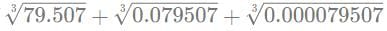is

Detailed Solution for Quantitative Aptitude - Test 14 - Question 18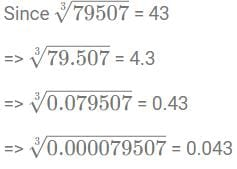=> 4.3 + 0.43 + 0.043 = 4.773

Quantitative Aptitude - Test 14 - Question 19

If √6 x √15 = x√10, then the value of x is

Detailed Solution for Quantitative Aptitude - Test 14 - Question 19

It is given that √6 x √15 = x√10

√6 x √15 = √(3x2) x √(3 x 5) = √(9 x 10) = 3 √10

Hence x = 3

Quantitative Aptitude - Test 14 - Question 20

If x + y = 15, then (x − 10)3 + (y − 5)3 is

Detailed Solution for Quantitative Aptitude - Test 14 - Question 20

given that x + y = 15

So rearranging the above equation

(x - 10 ) + (y - 5) =0

Now , x - 10 = -(y - 5)

On cubing both sides
(x − 10)3 = - (y − 5)3
And hence
(x − 10)3 + (y − 5)3 = 0

Quantitative Aptitude - Test 14 - Question 21

The value of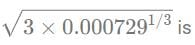Detailed Solution for Quantitative Aptitude - Test 14 - Question 21

We need to find value of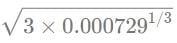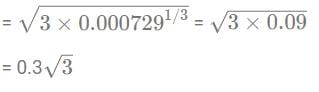= 0.519 ~ 0.52

Quantitative Aptitude - Test 14 - Question 22

If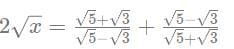Detailed Solution for Quantitative Aptitude - Test 14 - Question 22

It is given that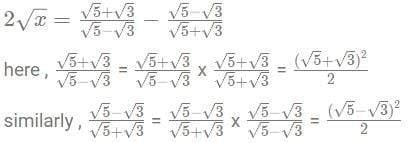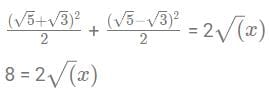x = 16

Quantitative Aptitude - Test 14 - Question 23

If a + 1/a = 1, then the value of a2 + 1/a2 is:

Detailed Solution for Quantitative Aptitude - Test 14 - Question 23

it is given that a + 1/a = 1

and we need to find value of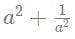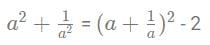= 12 - 2 = -1

Quantitative Aptitude - Test 14 - Question 24

The value of x when 5% of √2x is 0.01 will be:

Detailed Solution for Quantitative Aptitude - Test 14 - Question 24

It is given that 5% of √2x is 0.01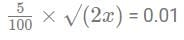x = 0.02

Quantitative Aptitude - Test 14 - Question 25

If a * b = 2a + 3b - ab, then the value of (3 * 5 + 5 * 3) is

Detailed Solution for Quantitative Aptitude - Test 14 - Question 25

For 3 * 5 put a = 3 and b = 5 in given equation
and for 5 * 3 put a = 5 and b = 3 in equation

## SSC CGL Tier 1 Mock Test Series

64 docs|118 tests
 Use Code STAYHOME200 and get INR 200 additional OFF Use Coupon Code
Information about Quantitative Aptitude - Test 14 Page
In this test you can find the Exam questions for Quantitative Aptitude - Test 14 solved & explained in the simplest way possible. Besides giving Questions and answers for Quantitative Aptitude - Test 14, EduRev gives you an ample number of Online tests for practice

## SSC CGL Tier 1 Mock Test Series

64 docs|118 tests